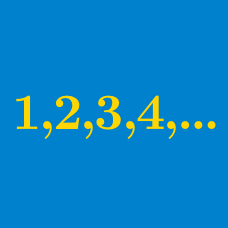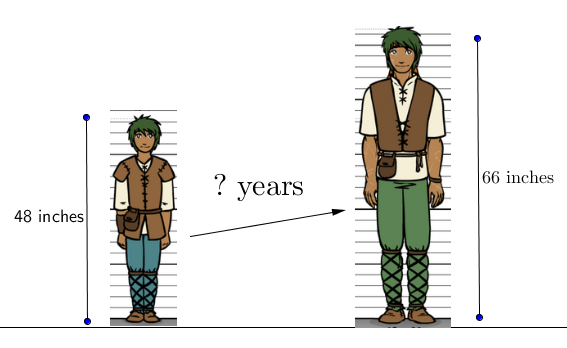Algebra

Finding the nth Term - Arithmetic Progressions

If the $4$ numbers

$14,\quad a,\quad 28,\quad b$

form an arithmetic progression in that order, what is the sum of $a$ and $b?$You love marbles and start collecting them. You have 2 marbles initially and add the same number of marbles to your store each day. If you have 17 marbles total after 5 days, then how many marbles did you add each day?

An arithmetic progression has first term $a_{1} = 2$ and common difference $d = 6$. What is the 5th term of the progression, $a_{5}$?A frog is on the bottom of an empty well $108\text{ m}$ deep. It climbs up the well $3\text{ m}$ during the daytime and slips down $1\text{ m}$ at nighttime. What is the number of days it takes for the frog to be out of the well?Suppose that when you are a teenager, your height increases at a steady rate of 3 inches every year. If your present height is 48 inches, then how many years will you take to grow to 66 inches tall?

×